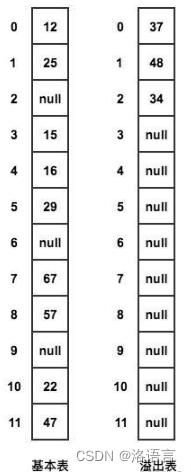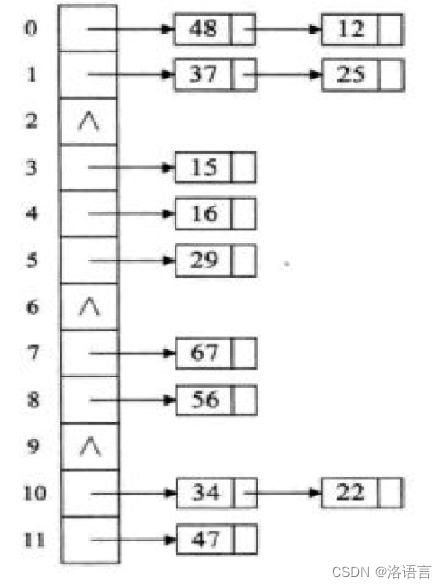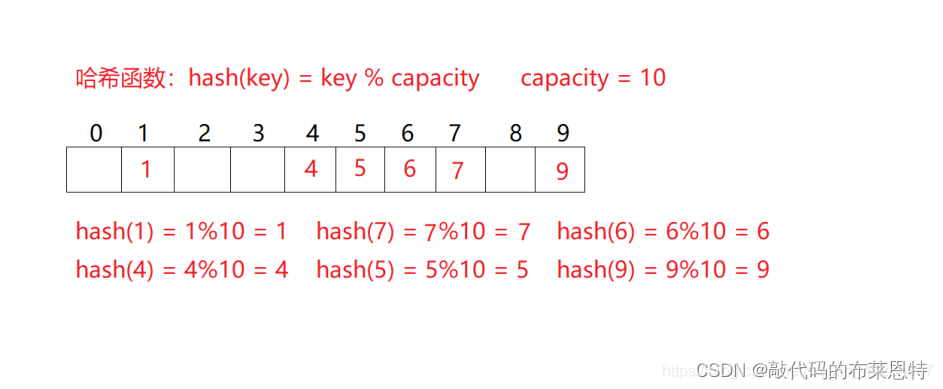+关注继续查看

# 哈希表概念

• 散列表，又称为哈希表（Hash table），采用散列技术将记录存储在一块连续的存储空间中。
• 在散列表中，我们通过某个函数f，使得存储位置 = f（关键字），这样我们可以不需要比较关键字就可获得需要的记录的存储位置。
• 散列技术的记录之间不存在什么逻辑关系，它只与关键字有关联。因此，散列主要是面向查找的存储结构。

# 哈希函数的构造

1. 计算简单
散列函数的计算时间不应该超过其他查找技术与关键字比较的时间。
2. 散列地址分布均匀
解决冲突最好的办法就是尽量让散列地址均匀地分布在存储空间中。
3. 保证存储空间的有效利用，并减少为处理冲突而耗费的时间。

## 保留余数法

f(key) = key mod p (p <= m)

public int hashFunc(int key){
return key % length;
}


# 哈希冲突问题以及解决方法

key1 ≠ key2，但是f（key1）= f（key2）

## 开放地址法

例子：
19 01 23 14 55 68 11 86 37



## 公共溢出区法## 链式地址法# 哈希表的填充因子

填充因子就是 ：表中已经填有元素的位置个数 / 哈希表长度

# 代码实现

public class HashTableDemo {
//哈希表每个位置链表的节点
class Node{
//关键字
int key;
String value;
Node next;
//无参构造
Node(){}
//有参构造
Node(int key, String value){
this.key = key;
this.value = value;
next = null;
}
//重写哈希表的equals()方法
public boolean equals(Node node){
if(this == node) return true;
else{
if(node == null) return false;
else{
return this.value == node.value && this.key == node.key;
}
}
}
}
//哈希表的长度
int length;
//哈希表存的键值对个数
int size;
//存储数据容器
Node table[];
//不指定初始化长度的无参构造
public HashTableDemo(){
length = 16;
size = 0;
table = new Node[length];
//为哈希表每一个位置初始化
for (int i = 0; i < length; i++) {
table[i] = new Node(i,null);
}
}
//指定初始化长度的有参构造
public HashTableDemo(int length){
this.length = length;
size = 0;
table = new Node[length];
for (int i = 0; i < length; i++) {
table[i] = new Node(i,null);
}
}
}

## 哈希函数

public int hashFunc(int key){
return key % length;
}


## 添加数据

1. 先通过哈希函数算出该键值对在table中的位置。
2. 遍历该处的链表的每一个节点，若发现某节点的key与传入的key相等，那么就更新此处的value。
3. 若未发现相等的key，那么在链表末尾添加新的节点.
4. 最后返回value。

public String put(int key, String value){
int index = hashFunc(key);
//保证cur2始终是cur的前一个节点。
Node cur = table[index].next;
Node cur2 = table[index];
while(cur != null){
if(cur.key == key){
cur.value = value;
return value;
}
cur = cur.next;
cur2 = cur2.next;
}
cur2.next = new Node(key, value);
size++;
return value;
}


## 删除数据

1. 先通过哈希函数算出该键值对在table中的位置。
2. 遍历该处的链表的每一个节点，若发现某节点的key与传入的key相等，那么就删除此节点，并返回它的value。
3. 若未发现相等的key，返回null。

public String remove(int key){
int index = hashFunc(key);
Node cur = table[index];
while(cur.next != null){
if(cur.next.key == key){
size--;
String value = cur.next.value;
cur.next = cur.next.next;
return value;
}
cur = cur.next;
}
return null;
}


## 判断哈希表是否为空

public boolean isEmpty(){
for(int i = 0; i < length; i++){
if(table[i].next != null)
return false;
}
return true;
}


## 遍历哈希表

public void print(){
for(int i = 0; i < length; i++){
Node cur = table[i];
System.out.printf("第%d条链表: ",i);
if(cur.next == null){
System.out.println("null");
continue;
}
cur = cur.next;
while(cur != null){
System.out.print(cur.key + "---"+ cur.value + "  ");
cur = cur.next;
}
System.out.println();
}
}


## 获得哈希表已存键值对个数

//返回哈希表已存数据个数
public int size(){
return size;
}【Java数据结构】哈希表——学习笔记

38 0【Java数据结构】Java对象的比较
【Java数据结构】Java对象的比较
81 056 0Java数据结构之单链表
1034 0【Java数据结构】栈
1105 0Java中的数据结构一览
Java的类库实在是很多，以至于很多人都不太了解，结果总是自己造轮子。 下面汇总了Java中的一些数据结构，加上一些实现的分析，同时备忘。
781 0Java中的数据结构一览
1467 0JAVA数据结构--链表

551 0
61

0